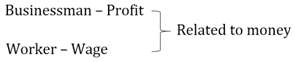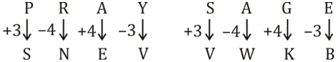# SSC Reasoning Ability Practice Questions (Day-74)

Dear Aspirants, The SSC Exams are going on at national level. Millions of candidates have eagerly prepared for the ongoing SSC exams such as CGL, CHSL, MTS, CPO, JE etc. SSC exams are usually conducted by the Staff Selection Commission to recruit the eligible candidates for various Central government departments. Mostly the selection process for all the SSC exams consists of the single or double phase of written examination based on the examination. Due to that, the written exam consisted of a certain syllabus and exam pattern. By following those regulations, the written exam has been conducted through an online mode. Based on the latest updated exam pattern and syllabus, here we have drafted the Reasoning Ability questions on a daily basis. So aspirants start your practice session with us and mould your skill in a perfect shape.

Start Quiz

Direction (1-2): In the following question, select the related word pair from the given alternatives.

1) Businessman : Profit : : ? : ?

(a) Doctor : Patient

(b) Worker : Wage

(c) Police : Criminal

(d) Court : Judge

2) In the following question, select the related word pair from the given alternatives.

Mathematics : Numbers : : ? : ?

(a) Geography : Chemicals

(b) Chemistry : Law of motion

(c) History : Events

(d) Economics : Dates

3) In the following question, select the related letters from the given alternatives.

N : R : : V : ?

(a) Z

(b) A

(c) Y

(d) B

4) In the following question, select the related letter pair from the given alternatives.

PRAY : SNEV : : ? : ?

(a) GREY : JPIU

(b) PONG : SPIB

(c) SAGE : VWKB

(d) LIST : ILVQ

Direction (5-6): In the following question, select the related number from the given alternatives.

5) 42 : 14 : : 56 : ?

(a) 18

(b) 41

(c) 48

(d) 65

6) 142 : 15 : : 234 : ?

(a) 45

(b) 39

(c) 33

(d) 47

Direction (7-8): In the following question, select the odd word from the given alternatives.

7)

(a) Earth

(b) Sun

(c) Mercury

(d) Jupiter

8) In the following question, select the odd word from the given alternatives.

(a) Eagle

(b) Crow

(c) Pigeon

(d) Ostrich

Direction (9-10): In the following question, select the odd letters from the given alternatives.

9)

(a) QS

(b) WY

(c) HK

(d) LN

10)

(a) FJEK

(b) UXTV

(c) IMHN

(d) PTOU

Answers :

1) Answer: B2) Answer: C

Number is related to Mathematics

Events is related to History

3) Answer: A

+4 Series

4) Answer: C5) Answer: B

(4 × 2) + (4 + 2) = 14

(5 × 6) + (5 + 6) = 41

6) Answer: C

(1 × 4 ×2 ) + (1 + 4 + 2) = 15

(2 × 3 × 4) + (2 + 3 + 4) = 33

7) Answer: B

Sun is not planet.

8) Answer: D

Ostrich cannot fly.

9) Answer: C

+2 series, except HK.

10) Answer: B

+4, –5, +6 series except UXTV.

 Check Here to View SSC CGL / CHSL 2021 Reasoning Ability Questions Day – 73 Day – 72 Day – 71
0 0 votes
Rating0 Comments
Inline Feedbacks
View all comments